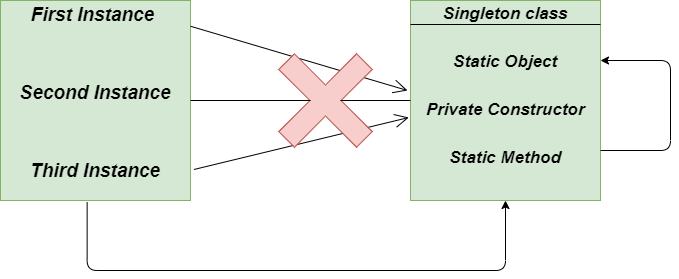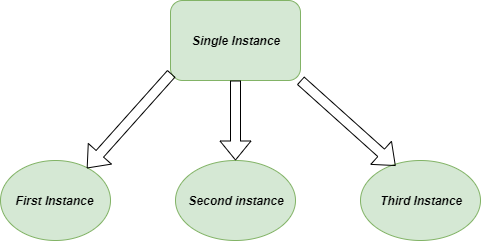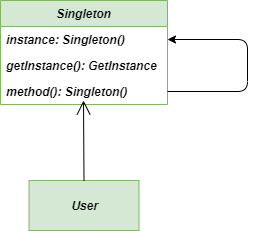Open in App
Not now

# Singleton Method – Python Design Patterns

• Difficulty Level : Basic
• Last Updated : 08 Jul, 2022

Prerequisite: Singleton Design pattern | Introduction

## What is Singleton Method in Python

Singleton Method is a type of Creational Design pattern and is one of the simplest design patterns00 available to us. It is a way to provide one and only one object of a particular type. It involves only one class to create methods and specify the objects.
Singleton Design Pattern can be understood by a very simple example of Database connectivity. When each object creates a unique Database Connection to the Database, it will highly affect the cost and expenses of the project. So, it is always better to make a single connection rather than making extra irrelevant connections which can be easily done by Singleton Design Pattern.singleton-pattern

Definition: The singleton pattern is a design pattern that restricts the instantiation of a class to one object.

Now let’s have a look at the different implementations of the Singleton Design pattern.

## Method 1: Monostate/Borg Singleton Design pattern

Singleton behavior can be implemented by Borg’s pattern but instead of having only one instance of the class, there are multiple instances that share the same state. Here we don’t focus on the sharing of the instance identity instead we focus on the sharing state.

## Python3

 `# Singleton Borg pattern``class` `Borg:` `    ``# state shared by each instance``    ``__shared_state ``=` `dict``()` `    ``# constructor method``    ``def` `__init__(``self``):` `        ``self``.__dict__ ``=` `self``.__shared_state``        ``self``.state ``=` `'GeeksforGeeks'` `    ``def` `__str__(``self``):` `        ``return` `self``.state`  `# main method``if` `__name__ ``=``=` `"__main__"``:` `    ``person1 ``=` `Borg()    ``# object of class Borg``    ``person2 ``=` `Borg()    ``# object of class Borg``    ``person3 ``=` `Borg()    ``# object of class Borg` `    ``person1.state ``=` `'DataStructures'`  `# person1 changed the state``    ``person2.state ``=` `'Algorithms'`     `# person2 changed the state` `    ``print``(person1)    ``# output --> Algorithms``    ``print``(person2)    ``# output --> Algorithms` `    ``person3.state ``=` `'Geeks'`  `# person3 changed the``    ``# the shared state` `    ``print``(person1)    ``# output --> Geeks``    ``print``(person2)    ``# output --> Geeks``    ``print``(person3)    ``# output --> Geeks`

Output:

```Algorithms
Algorithms
Geeks
Geeks
Geeks```Singleton-Design-pattern

### Double Checked Locking Singleton Design pattern

It is easy to notice that once an object is created, the synchronization of the threading is no longer useful because now the object will never be equal to None and any sequence of operations will lead to consistent results.
So, when the object will be equal to None, then only we will acquire the Lock on the getInstance method.

## Python3

 `# Double Checked Locking singleton pattern``import` `threading`  `class` `SingletonDoubleChecked(``object``):` `    ``# resources shared by each and every``    ``# instance` `    ``__singleton_lock ``=` `threading.Lock()``    ``__singleton_instance ``=` `None` `    ``# define the classmethod``    ``@classmethod``    ``def` `instance(``cls``):` `        ``# check for the singleton instance``        ``if` `not` `cls``.__singleton_instance:``            ``with ``cls``.__singleton_lock:``                ``if` `not` `cls``.__singleton_instance:``                    ``cls``.__singleton_instance ``=` `cls``()` `        ``# return the singleton instance``        ``return` `cls``.__singleton_instance`  `# main method``if` `__name__ ``=``=` `'__main__'``:` `    ``# create class X``    ``class` `X(SingletonDoubleChecked):``        ``pass` `    ``# create class Y``    ``class` `Y(SingletonDoubleChecked):``        ``pass` `    ``A1, A2 ``=` `X.instance(), X.instance()``    ``B1, B2 ``=` `Y.instance(), Y.instance()` `    ``assert` `A1 ``is` `not` `B1``    ``assert` `A1 ``is` `A2``    ``assert` `B1 ``is` `B2` `    ``print``(``'A1 : '``, A1)``    ``print``(``'A2 : '``, A2)``    ``print``(``'B1 : '``, B1)``    ``print``(``'B2 : '``, B2)`

Output:

```A1 :  __main__.X object at 0x02EA2590
A2 :  __main__.X object at 0x02EA2590
B1 :  __main__.Y object at 0x02EA25B0
B2 :  __main__.Y object at 0x02EA25B0```

## Creating a singleton in Python

In the classic implementation of the Singleton Design pattern, we simply use the static method for creating the getInstance method which has the ability to return the shared resource. We also make use of the so-called Virtual private Constructor to raise the exception against it although it is not much required.

## Python3

 `# classic implementation of Singleton Design pattern``class` `Singleton:` `    ``__shared_instance ``=` `'GeeksforGeeks'` `    ``@staticmethod``    ``def` `getInstance():``        ``"""Static Access Method"""``        ``if` `Singleton.__shared_instance ``=``=` `'GeeksforGeeks'``:``            ``Singleton()``        ``return` `Singleton.__shared_instance` `    ``def` `__init__(``self``):``        ``"""virtual private constructor"""``        ``if` `Singleton.__shared_instance !``=` `'GeeksforGeeks'``:``            ``raise` `Exception(``"This class is a singleton class !"``)``        ``else``:``            ``Singleton.__shared_instance ``=` `self`  `# main method``if` `__name__ ``=``=` `"__main__"``:` `    ``# create object of Singleton Class``    ``obj ``=` `Singleton()``    ``print``(obj)` `    ``# pick the instance of the class``    ``obj ``=` `Singleton.getInstance()``    ``print``(obj)`

Output:

``` __main__.Singleton object at 0x014FFE90
__main__.Singleton object at 0x014FFE90```

### Class diagram

Class Diagram of Singleton Design Patternsingleton-class-diagram

### Advantages of using the Singleton Method:

1. Initializations: An object created by the Singleton method is initialized only when it is requested for the first time.
3. Count of instances: In singleton, method classes can’t have more than one instance

### Disadvantages of using the Singleton Method:

1. Multithread Environment: It’s not easy to use the singleton method in a multithread environment, because we have to take care that the multithread wouldn’t create a singleton object several times.
2. Single responsibility principle: As the Singleton method is solving two problems at a single time, it doesn’t follow the single responsibility principle.
3. Unit testing process: As they introduce the global state to the application, it makes the unit testing very hard.

### Applicability

1. Controlling over global variables: In the projects where we specifically need strong control over the global variables, it is highly recommended to use Singleton Method
2. Daily Developers use: Singleton patterns are generally used in providing the logging, caching, thread pools, and configuration settings and are often used in conjunction with Factory design patterns.

My Personal Notes arrow_drop_up